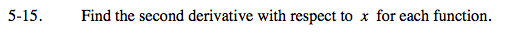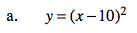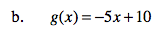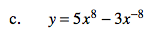### Home > CALC > Chapter 5 > Lesson 5.1.2 > Problem5-15

5-15.This is the equation of a parabola. The 2nd derivative represents the concavity of the original equation.
Is y = (x −10)² concave up, concave down or neither?g(x) is a linear equation.
g ''(x) represents its concavity.
Is a straight line concave up, concave down or neither?Use the Power Rule.